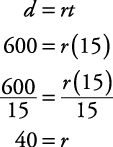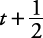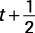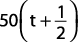## Motion Problems

Here are some examples for solving motion problems.

##### Example 1

How long will it take a bus traveling 72 km/hr to go 36 kms?

First circle what you're trying to find— how long will it take (time). Motion problems are solved by using the equationTherefore, simply plug in: 72 km/hr is the rate (or speed) of the bus, and 36 km is the distance.Therefore, it will take one‐half hour for the bus to travel 36 km at 72 km/hr.

##### Example 2

How fast in miles per hour must a car travel to go 600 miles in 15 hours?

First, circle what you must find— how fast (rate). Now, using the equation d = rt, simply plug in 600 for distance and 15 for time.So, the rate is 40 miles per hour.

##### Example 3

Mrs. Benevides leaves Burbank at 9 a.m. and drives west on the Ventura Freeway at an average speed of 50 miles per hour. Ms. Twill leaves Burbank at 9:30 a.m. and drives west on the Ventura Freeway at an average speed of 60 miles per hour. At what time will Ms. Twill overtake Mrs. Benevides, and how many miles will they each have gone?

First, circle what you are trying to find— at what time and how many miles. Now, let t stand for the time Ms. Twill drives before overtaking Mrs. Benevides. Then Mrs. Benevides drives forhours before being overtaken. Next, set up the following chart.

rate r

x

time t

=

distance d

Ms. Twill

60 mph

t

60 t

Mrs. Benevides

50 mphBecause each travels the same distance,Ms. Twill overtakes Mrs. Benevides after 2.5 hours of driving. The exact time can be figured out by using Ms. Twill's starting time: 9:30 + 2:30 = 12 noon. Since Ms. Twill has traveled for 2.5 hours at 60 mph, she has traveled 2.5 × 60, which is 150 miles. So, Mrs. Benevides is overtaken at 12 noon, and each has traveled 150 miles.